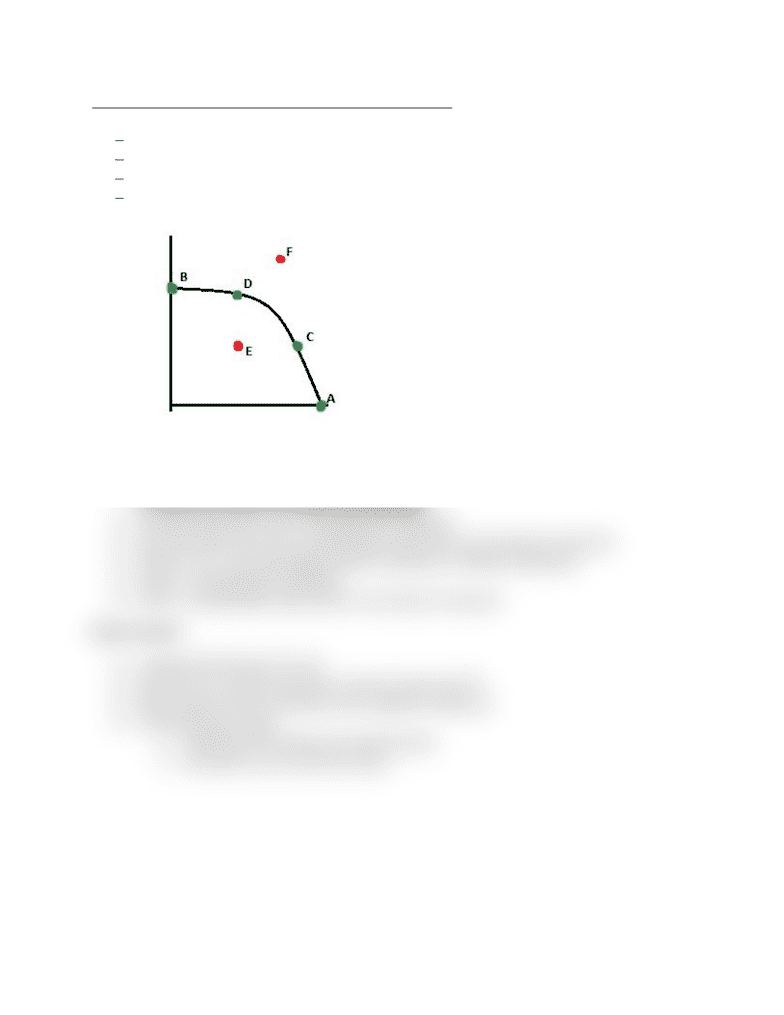# MGEA02H3 Lecture Notes - Lecture 2: Opportunity Cost

175 views1 pagesMGEA02 – Lecture 2 – Production Possibilities Frontier (PPF)
Economic resources are scarce the PPF shows the limit to what can be produced
PPF is a set of all possible outcomes of production
PPF usually has a bowed-out shape; this is because the opportunity cost (dY/dX) is decreasing.
PPF can be a straight line
Opportunity cost (of X) = OCx = (-dY/dX)
Opportunity cost (of Y) = OCy = (-dX/dY)
Refer to diagram on right
Graph shows the outer limit that can be produced with the amount of limited resources
Any point on the curve is efficient and attainable
Any point below the curve is attainable but inefficient
Any point above the curve is unattainable unless there is an increase in resources
Points, A, B, C, D are all possible choices to produce at. Efficient production
Point E. Is attainable but inefficient
Point F is unattainable unless there is an increase in resources
Value Function
Consider the function V=3x +6y
Each unit of Y is worth two times as much as each unit of X
More of good Y that is produced, more of good X is given up
To find maximum value
oSubstitute PPF function into value function
oSet equal to zero to find max value
Unlock document

This preview shows half of the first page of the document.
Unlock all 1 pages and 3 million more documents.

## Document Summary

Mgea02 lecture 2 production possibilities frontier (ppf) Economic resources are scarce the ppf shows the limit to what can be produced. Ppf is a set of all possible outcomes of production. Ppf usually has a bowed-out shape; this is because the opportunity cost (dy/dx) is decreasing. Opportunity cost (of x) = ocx = (-dy/dx) Opportunity cost (of y) = ocy = (-dx/dy) Graph shows the outer limit that can be produced with the amount of limited resources. Any point on the curve is efficient and attainable. Any point below the curve is attainable but inefficient. Any point above the curve is unattainable unless there is an increase in resources. Points, a, b, c, d are all possible choices to produce at. Point f is unattainable unless there is an increase in resources. Each unit of y is worth two times as much as each unit of x.